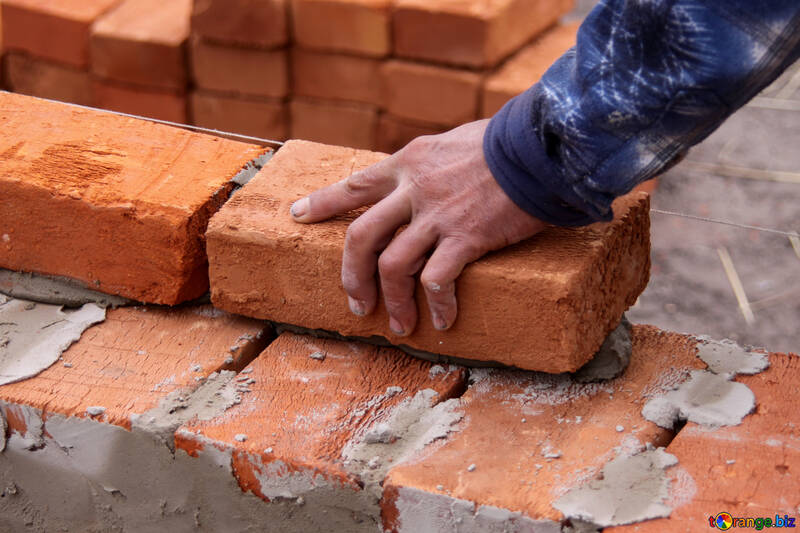# Mortar Calculator

Created by Maria Kluziak
Reviewed by Bogna Szyk and Jack Bowater
Last updated: Jan 04, 2023

This mortar calculator is a simple tool that will come in handy for any wall construction project! If you've ever had to ask yourself: "How much mortar do I need?", you're in the right place. Use this calculator to find out the amount of materials you should buy for your project. Read on if you want to find out how many bags of mortar per concrete block or brick are typically needed, and enjoy having the calculations done for you in no time!

## How to use the mortar calculator?

Our calculator is quite a simple tool, and using it should take you no time. All you need to do is:

1. Pick whether you're using bricks or blocks
2. Input the number of bricks or blocks,
• If you don't yet know the number, don't worry! You can use our concrete block and brick calculators (linked in this mortar calculator) to easily find out how many of each are needed to complete your project.
3. Input the yield of one bag of the mortar you are using. Yield depends on the size of the blocks or bricks and the mortar's type. If you're not sure what the yield is for your particular bag/block combination, don't worry - you can refer to the table we have prepared later in this article.
4. Read the estimated number of mortar bags you should buy at the bottom of the mortar calculator!

Please note that calculating how many mortar bags per block are needed by hand will most likely give you number with a decimal place. As bags are not sold in parts, this tool takes this into account, and rounds the result up to the nearest whole number. If you want to do it yourself, you can use our round to nearest integer calculator.

As with most construction works, it's recommended that you buy a little more materials than you need - just in case something unexpected happens, it's better to be prepared in advance than waste time and money driving back and forth) to the store. Getting an additional 10% is a common practice; feel free to use our percentage calculator if you need to check how much excess material you need.

## What's the yield of a mortar bag?

To answer the question "How much mortar do I need?", you need to know the yield of one mortar bag. The following table will provide you with that information for some of the more common block and brick sizes. While it's impossible to state the exact number due, to differences in design, site conditions, etc., values within these intervals are generally a safe bet to answer how many bags of mortar per block are needed.

Block/Brick size

$80\ \mathrm{lb}$ bag

$3,000\ \mathrm{lb}$ bag

Modular bricks

$40$ - $45$

$1,500$ - $1,675$

Queen size bricks

$34$ - $39$

$1,275$ - $1,450$

King size bricks

$28$ - $33$

$1,050$ - $1,225$

Utility bricks

$23$ - $28$

$850$ - $1,050$

4 inch blocks

$15$ - $17$

$575$ - $650$

6 inch blocks

$12$ - $14$

$475$ - $525$

8 inch blocks

$11$ - $13$

$450$ - $500$

10 inch blocks

$11$ - $13$

$450$ - $500$

12 inch blocks

$10$ - $12$

$375$ - $425$

We made two specific tools for your construction needs: check our brick calculator and concrete block calculator to calculate how many pieces you need!

## How to calculate how much mortar do I need?

If you want to calculate the number of mortar bags on your own, go for it! Provided that you already know the number of bricks/blocks you need for your project, the process is really simple, and uses only one short equation:

bags of mortar = number of blocks or bricks / a single bag's yield

As the result is rarely an integer, remember to round it up!

If you need to work out the number of blocks or bricks, feel free to use our block and brick calculators.

Let's go through a quick example. Say you're building a wall from $5000$ utility bricks, and you can only buy $80\ \mathrm{lb}$ mortar bags. According to the table above, the yield per bag is between $23$ and $28$, so let's stick with $24$. So:

$\frac{5,\!000}{24}=208.3$

After rounding up, we find that, for such a project you would need 209 80lb mortar bags.Maria Kluziak
Calculate mortar for:
bricks
If you don't know the number of bricks, use our brick calculator.
Number of bags calculation
# of bricks
Yield of one mortar bag
Mortar bags
Weight calculation
Single bag's weight
lb
Total weight
lb
People also viewed…

### Car crash force

With this car crash calculator, you can find out how dangerous car crashes are.

### Concrete block fill

This concrete block fill calculator will help you determine how much concrete or mortar is needed to fill the void spaces inside a hollow concrete block wall of any size.

### Pipe weight

This pipe weight calculator can help you quickly determine the weight of a pipe, whether made of metal (like steel, brass, or aluminum) or plastic (like PVC).

### Social Media Time Alternatives

Check what you could have accomplished if you get out of your social media bubble.